Next: Tensors and pseudo-tensors Up: Relativity and electromagnetism Previous: Gauge invariance

## Retarded potentials

We already know the solutions to Eqs. (1439) and (1440). They take the form (see Sect. 4.9)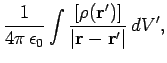(1451)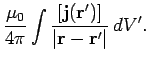(1452)

The above equations can be combined to form the solution of the 4-vector wave equation (1442),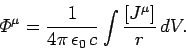(1453)

Here, the components of the 4-potential are evaluated at some eventin space-time,is the distance of the volume element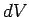from, and the square brackets indicate that the 4-current is to be evaluated at the retarded time: i.e., at a time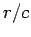before.

But, does the right-hand side of Eq. (1453) really transform as a contravariant 4-vector? This is not a trivial question, since volume integrals in 3-space are not, in general, Lorentz invariant due to the length contraction effect. However, the integral in Eq. (1453) is not a straightforward volume integral, because the integrand is evaluated at the retarded time. In fact, the integral is best regarded as an integral over events in space-time. The events which enter the integral are those which intersect a spherical light wave launched from the eventand evolved backwards in time. In other words, the events occur before the event, and have zero interval with respect to. It is clear that observers in all inertial frames will, at least, agree on which events are to be included in the integral, since both the interval between events, and the absolute order in which events occur, are invariant under a general Lorentz transformation.

We shall now demonstrate that all observers obtain the same value of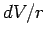for each elementary contribution to the integral. Suppose thatand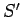are two inertial frames in the standard configuration. Let unprimed and primed symbols denote corresponding quantities inand, respectively. Let us assign coordinates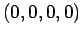to, and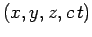to the retarded event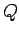for whichandare evaluated. Using the standard Lorentz transformation, (1346)-(1349), the fact that the interval between eventsandis zero, and the fact that bothand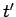are negative, we obtain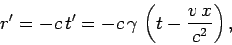(1454)

where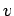is the relative velocity between framesand,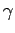is the Lorentz factor, and, etc. It follows that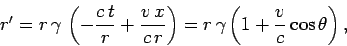(1455)

whereis the angle (in 3-space) subtended between the line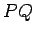and the-axis.

We now know the transformation for. What about the transformation for? We might be tempted to set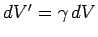, according to the usual length contraction rule. However, this is incorrect. The contraction by a factoronly applies if the whole of the volume is measured at the same time, which is not the case in the present problem. Now, the dimensions ofalong the- and-axes are the same in bothand, according to Eqs. (1346)-(1349). For the-dimension these equations give. The extremities of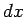are measured at times differing by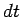, where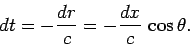(1456)

Thus,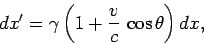(1457)

giving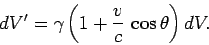(1458)

It follows from Eqs. (1455) and (1458) that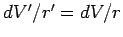. This result will clearly remain valid even whenandare not in the standard configuration.

Thus,is an invariant and, therefore,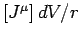is a contravariant 4-vector. For linear transformations, such as a general Lorentz transformation, the result of adding 4-tensors evaluated at different 4-points is itself a 4-tensor. It follows that the right-hand side of Eq. (1453) is indeed a contravariant 4-vector. Thus, this 4-vector equation can be properly regarded as the solution to the 4-vector wave equation (1442).Next: Tensors and pseudo-tensors Up: Relativity and electromagnetism Previous: Gauge invariance
Richard Fitzpatrick 2006-02-02